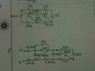# Find Equivalent Resistance Across Rab: A Geometric Conundrum

• Digdug12
In summary, the left hand resistance is R(L) // R(R), the right hand resistance is R(R) // R(L) and the total resistance across AB is R(AB) // R(L+R+R).

## Homework Statement

Find the equivalent resistance across Rab

## Homework Equations

Series: Req=R1+R2...Rn
Parallel Req=(R1 * R2 *...Rn) / (R1 + R2 +...Rn)

## The Attempt at a Solution

its the geometry that is throwing me off in this problem.
Starting on the left side,

I want to put 45 & 15 in series, then put that eq. resistance in parallel with the 40ohms and then that total resistance in series with the 6ohms, but I don't know what to do with the 1.5 by node A.

Same thing for the right side, 3 & 5 in series, then that in parallel with 12, that in series with 5.2, but then that in series with 1ohm by node B?

And then do I sum both of those Req's for the total Resistance across AB?

The 2nd problem.. I don't even know where to begin.

#### Attachments

•ECENhelp1.jpg
32.7 KB · Views: 351

I want to put 45 & 15 in series, then put that eq. resistance in parallel with the 40ohms and then that total resistance in series with the 6ohms
, but I don't know what to do with the 1.5 by node A.

Same thing for the right side, 3 & 5 in series, then that in parallel with 12, that in series with 5.2, but then that in series with 1ohm by node B?
Find left hand resistance R(L) and right hand resistance R(R)
Now find R(L) // R(R).
Finally, add all the three resistances to et R(AB)

It helps me to re-draw the circuit: start with node A at the top of your paper. Draw a vertical line extending downward. The only resistor connected directly to node A is the 2.5 ohm resistor; draw it in (vertically) and extend another vertical line downward. From here, the circuit splits, so draw a horizontal line off the bottom of your last line. There are 3 resistors that connect directly to the bottom of the 2.5 ohm resistor (3 ohm, 6 ohm and 26 ohm), so draw 3 vertical lines downward off the horizontal line you just drew; connect each of the 3 resistors to each of those lines and continue vertical lines below each.

Continue in this fashion, making sure not to re-use any resistors, until you reach node B (and have accounted for all the resistors).

This technique makes it easier to see how the components relate to each other so you can pick out the parallel and series combinations.